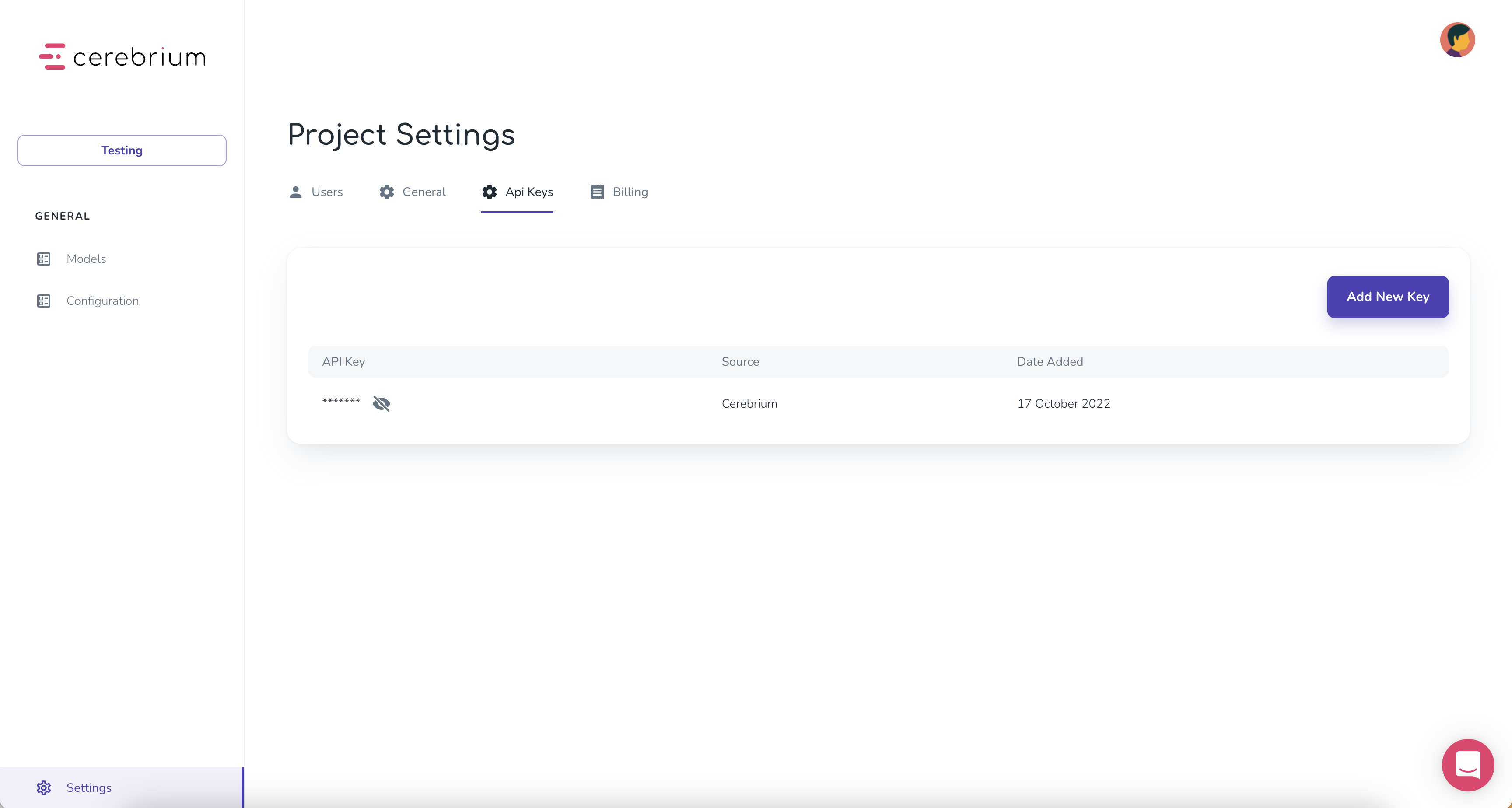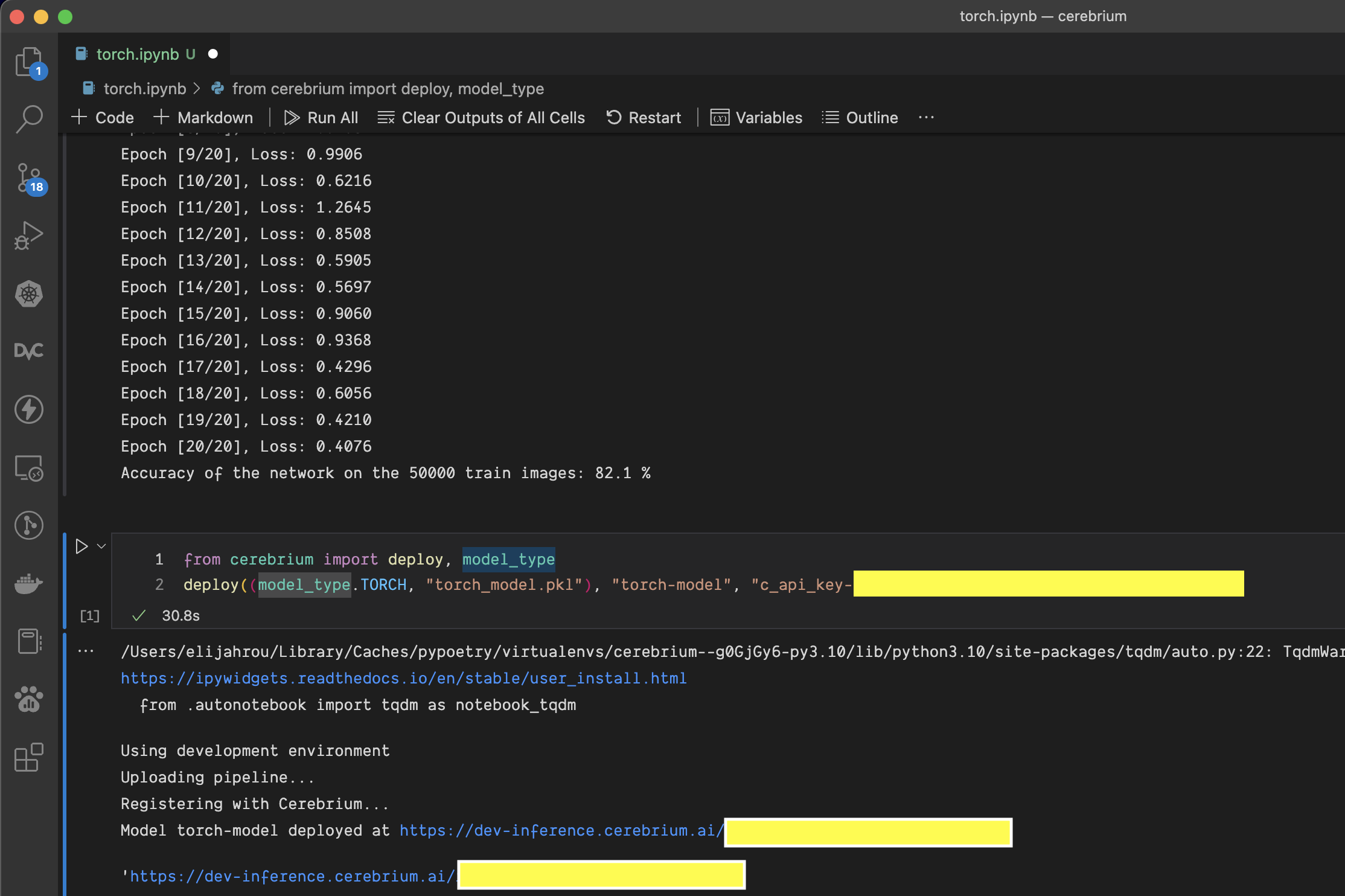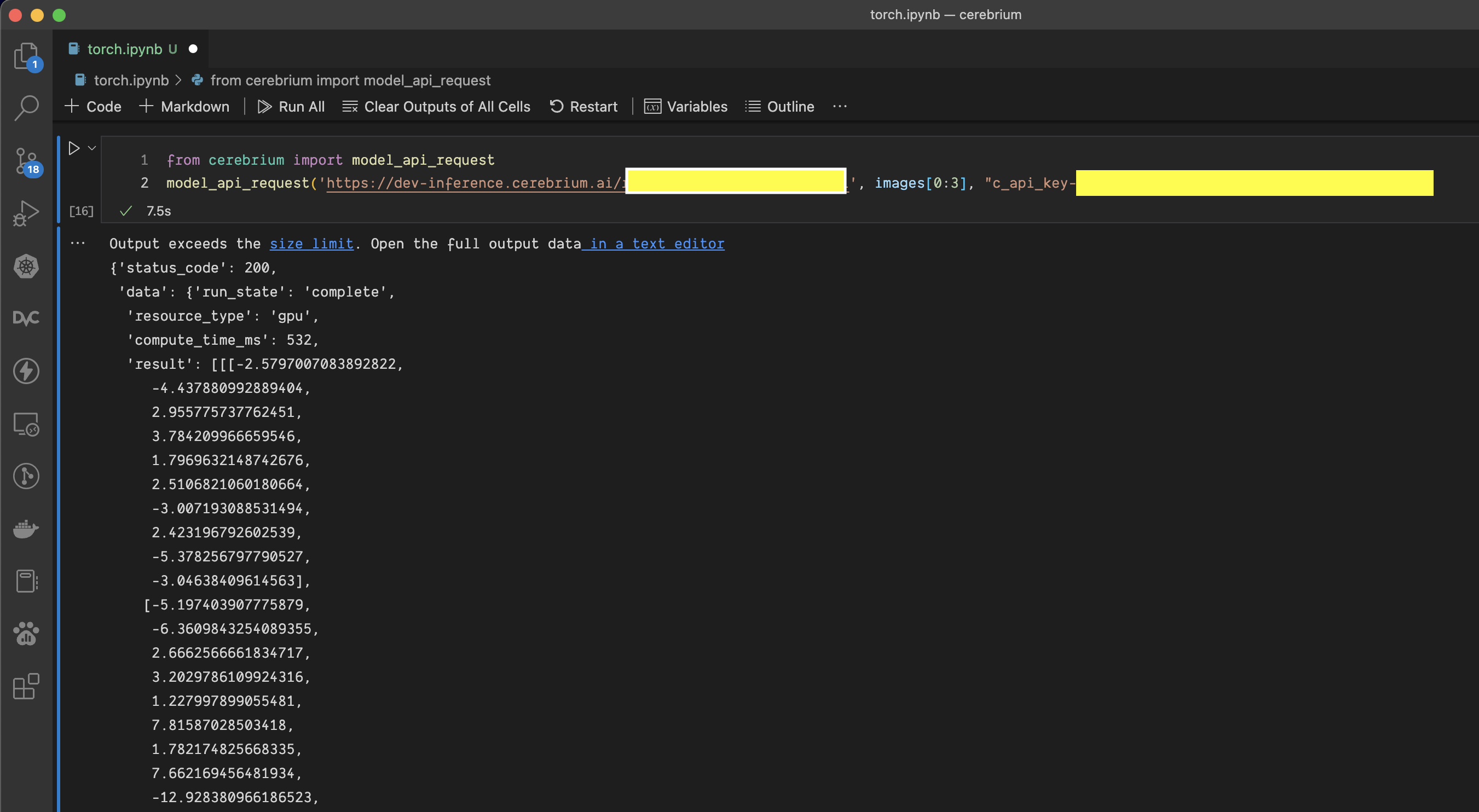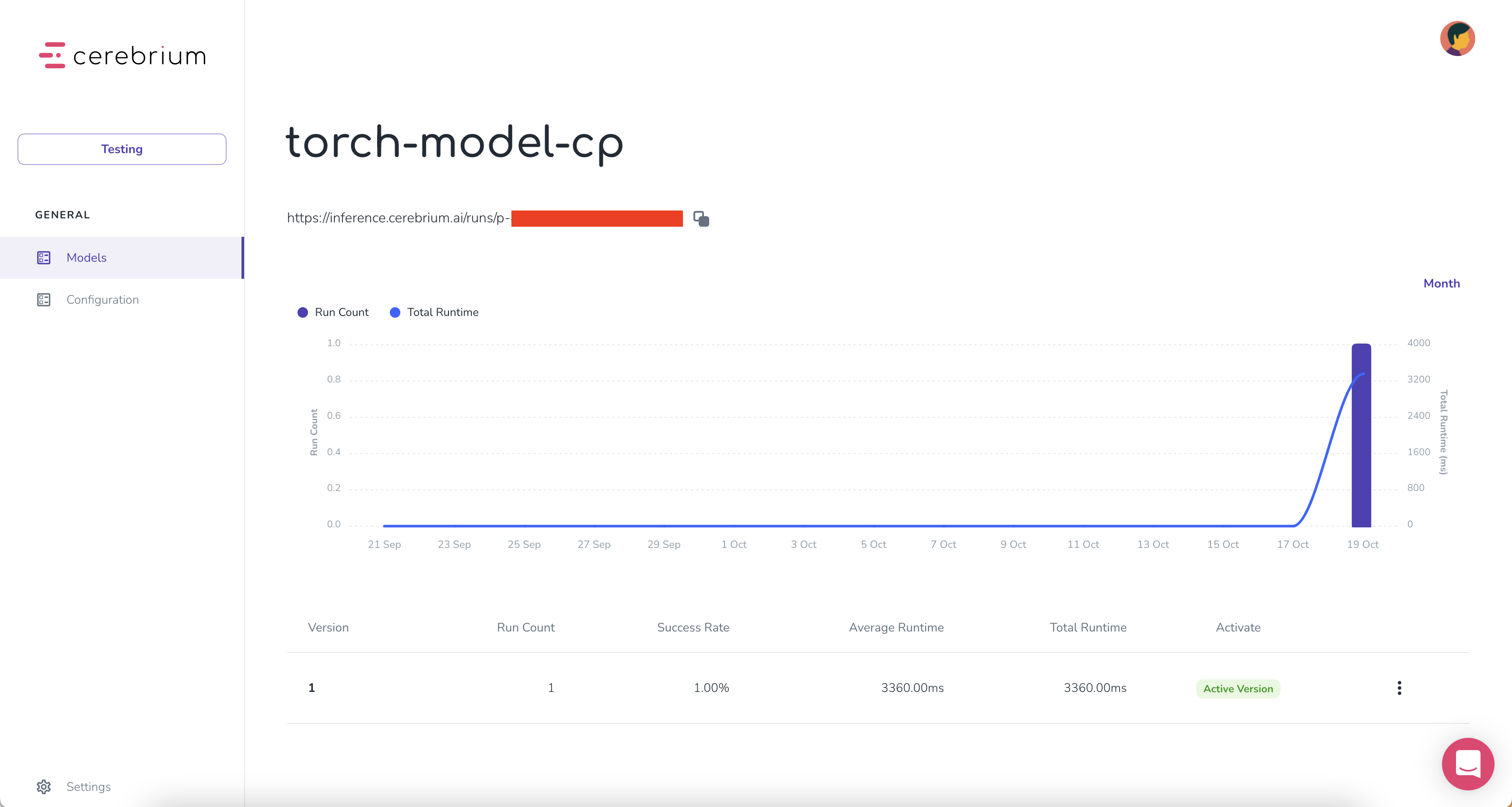## ​Intro

By the end of this guide you’ll have an API endpoint that can handle any scale of traffic by running inference on serverless CPU’s/GPU’s.

## ​Project set up

Before building you need to set up a Cerebrium account. This is as simple as starting a new Project in Cerebrium and copying the API key. This will be used to authenticate all calls for this project.

### ​Create a project

1. Go to dashboard.cerebrium.ai
3. Navigate to Settings page
4. Navigate to the API Keys tab
5. You should see an API key with the source “Cerebrium”. Click the eye icon to display it. It will be in the format: c_api_key-xxx### ​Develop model

Now navigate to where your model code is stored. This could be in a notebook or in a plain .py file.

Install the Cerebrium framework running the following command in your notebook or terminal

``````pip install cerebrium
``````

Copy and paste our code below. This creates a simple Convolutional Neural Network. This code could be replaced by any Pytorch model. Make sure you have the required libraries installed.

``````import torch
import torch.nn as nn
import torchvision
import torchvision.transforms as transforms

batch_size = 64
num_classes = 10
learning_rate = 0.001
num_epochs = 2

device = torch.device('cuda' if torch.cuda.is_available() else 'cpu')

# Use transforms.compose method to reformat images for modeling,
# and save to variable all_transforms for later use
all_transforms = transforms.Compose([transforms.Resize((32,32)),
transforms.ToTensor(),
transforms.Normalize(mean=[0.4914, 0.4822, 0.4465],
std=[0.2023, 0.1994, 0.2010])
])
# Create Training dataset
train_dataset = torchvision.datasets.CIFAR10(root = './data',
train = True,
transform = all_transforms,

# Create Testing dataset
test_dataset = torchvision.datasets.CIFAR10(root = './data',
train = False,
transform = all_transforms,

# Instantiate loader objects to facilitate processing
batch_size = batch_size,
shuffle = True)

batch_size = batch_size,
shuffle = True)

# Create Neural Network
class ConvNeuralNet(nn.Module):
def __init__(self, num_classes):
super(ConvNeuralNet, self).__init__()
self.conv_layer1 = nn.Conv2d(in_channels=3, out_channels=32, kernel_size=3)
self.conv_layer2 = nn.Conv2d(in_channels=32, out_channels=32, kernel_size=3)
self.max_pool1 = nn.MaxPool2d(kernel_size = 2, stride = 2)

self.conv_layer3 = nn.Conv2d(in_channels=32, out_channels=64, kernel_size=3)
self.conv_layer4 = nn.Conv2d(in_channels=64, out_channels=64, kernel_size=3)
self.max_pool2 = nn.MaxPool2d(kernel_size = 2, stride = 2)

self.fc1 = nn.Linear(1600, 128)
self.relu1 = nn.ReLU()
self.fc2 = nn.Linear(128, num_classes)

def forward(self, x):
out = self.conv_layer1(x)
out = self.conv_layer2(out)
out = self.max_pool1(out)

out = self.conv_layer3(out)
out = self.conv_layer4(out)
out = self.max_pool2(out)

out = out.reshape(out.size(0), -1)

out = self.fc1(out)
out = self.relu1(out)
out = self.fc2(out)
return out

model = ConvNeuralNet(num_classes)

# Create loss function and optimizer
criterion = nn.CrossEntropyLoss()

optimizer = torch.optim.SGD(model.parameters(), lr=learning_rate, weight_decay = 0.005, momentum = 0.9)

# Train our model
for epoch in range(num_epochs):
for i, (images, labels) in enumerate(train_loader):
images = images.to(device)
labels = labels.to(device)

outputs = model(images)
loss = criterion(outputs, labels)

loss.backward()
optimizer.step()

print('Epoch [{}/{}], Loss: {:.4f}'.format(epoch+1, num_epochs, loss.item()))

correct = 0
total = 0
images = images.to(device)
labels = labels.to(device)
outputs = model(images)
_, predicted = torch.max(outputs.data, 1)
total += labels.size(0)
correct += (predicted == labels).sum().item()

print('Accuracy of the network on the {} train images: {} %'.format(50000, 100 * correct / total))

## Save with Cloudpickle
import cloudpickle
with open("torch_model.pkl", "wb") as f:
cloudpickle.dump(model, f)

## Save with TorchScript
scripted_model = torch.jit.script(model)
scripted_model.save("torch_model.pt")
``````

In the last line of code, there are two ways you can save the model. This is all you need to deploy your model to Cerebrium! You can then import the `deploy()` function from the Cerebrium framework. I used the CloudPickle function to save my model below.

``````from cerebrium import deploy, model_type

endpoint = deploy((model_type.TORCH, "torch_model.pkl"), "torch-model-cp", "<API_KEY>")
``````Your model is now deployed and ready for inference all in under 10 seconds! Navigate to the dashboard and on the Models page you will see your model.

You can run inference using `curl` or the `model_api_request()` python helper function. The helper function is useful for large data.

``````curl --location --request POST '<ENDPOINT>' \
--data-raw '[<DATA>]'
``````
``````from cerebrium import model_api_request

response = model_api_request("<ENDPOINT_URL", images, "<API_KEY>")
print(response["data"])
``````Navigate back to the dashboard and click on the name of the model you just deployed. You will see an API call was made and the inference time. From your dashboard you can monitor your model, roll back to previous versions and see traffic.With one line of code, your model was deployed in seconds with automatic versioning, monitoring and the ability to scale based on traffic spikes. Try deploying your own model now or check out our other frameworks.